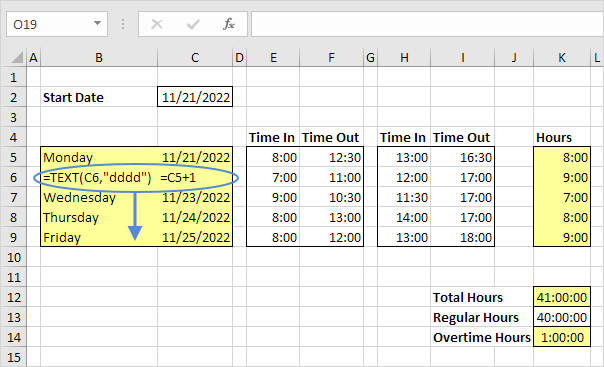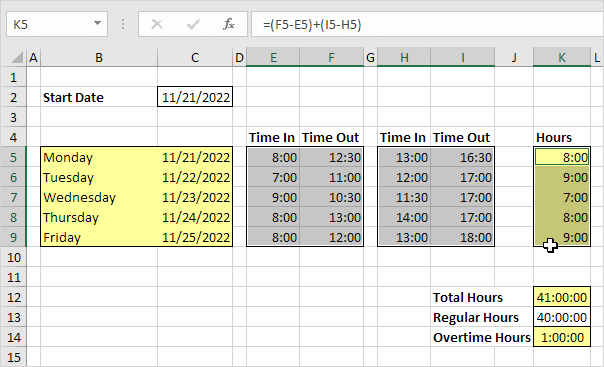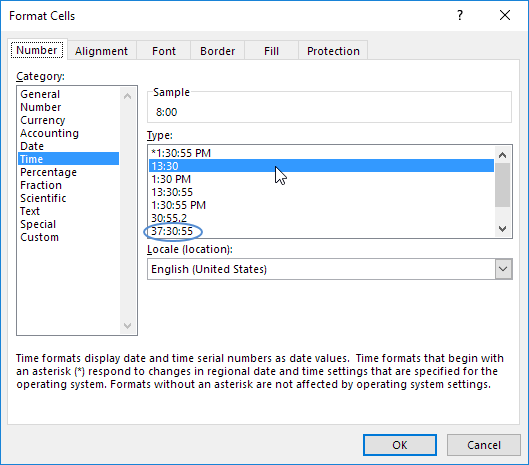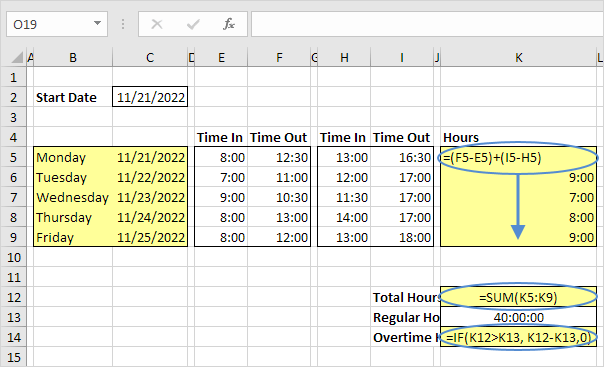# Time Sheet in Excel

This example teaches you how to create a simple timesheet calculator in Excel. Cells that contain formulas are colored light yellow. If you are in a hurry, simply download the Excel file.

1. To automatically calculate the next 4 days and dates when you enter a start date, use the formulas below.2. Select the cells containing the times.3. Right click, click Format Cells, and select the right Time format. Use the circled format for cell K12, K13 and K14.4. To automatically calculate the hours worked each day, the total hours and the overtime hours, use the formulas below.Go to Next Chapter: Data Validation